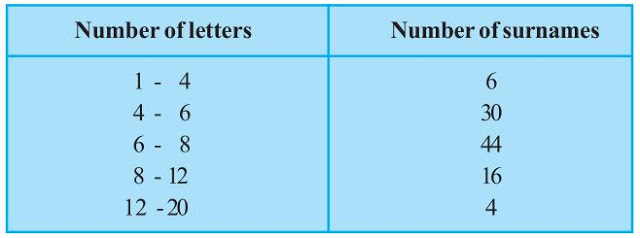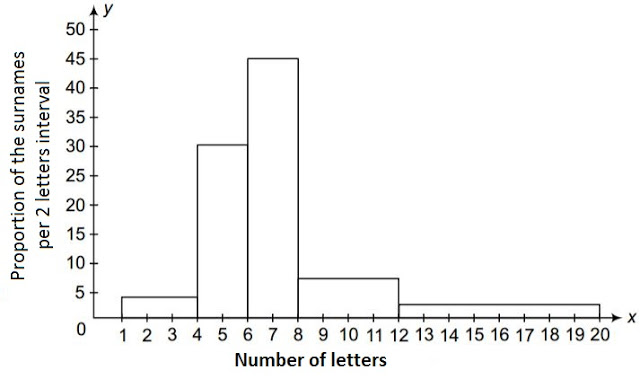# 100 surnames were randomly picked up from a local telephone directory and a frequency distribution of the number of letters in the English alphabet in the surnames was found as follows:(i) Draw a histogram to depict the given information. (ii) Write the class interval in which the maximum number of surnames lie.Solution

(i) The width of the class intervals in the given data is varying in nature.

Also, The area of a rectangle is proportional to the frequencies in the histogram.

Now, the proportion of children per year can be calculated as shown in the table below.

 Number of letters Number of surnames Width of class Length of rectangle 1-4 6 3 (6/3)×2 = 4 4-6 30 2 (30/2)×2 = 30 6-8 44 2 (44/2)×2 = 44 8-12 16 4 (16/4)×2 = 8 12-20 4 8 (4/8)×2 = 1(ii) 6-8 is the class interval in which the maximum number of surnames lie.